# Fraction calculator

The calculator performs basic and advanced operations with fractions, expressions with fractions combined with integers, decimals, and mixed numbers. It also shows detailed step-by-step information about the fraction calculation procedure. Solve problems with two, three, or more fractions and numbers in one expression.

## Result:

### 1/3 + 2/6 = 2/3 ≅ 0.6666667

Spelled result in words is two thirds.

### How do you solve fractions step by step?

1. Add: 1/3 + 2/6 = 1 · 2/3 · 2 + 2/6 = 2/6 + 2/6 = 2 + 2/6 = 4/6 = 2 · 2/2 · 3 = 2/3
For adding, subtracting, and comparing fractions, it is suitable to adjust both fractions to a common (equal, identical) denominator. The common denominator you can calculate as the least common multiple of both denominators - LCM(3, 6) = 6. In practice, it is enough to find the common denominator (not necessarily the lowest) by multiplying the denominators: 3 × 6 = 18. In the following intermediate step, cancel by a common factor of 2 gives 2/3.
In other words - one third plus two sixths = two thirds.

#### Rules for expressions with fractions:

Fractions - simply use a forward slash between the numerator and denominator, i.e., for five-hundredths, enter 5/100. If you are using mixed numbers, be sure to leave a single space between the whole and fraction part.
The slash separates the numerator (number above a fraction line) and denominator (number below).

Mixed numerals (mixed fractions or mixed numbers) write as integer separated by one space and fraction i.e., 1 2/3 (having the same sign). An example of a negative mixed fraction: -5 1/2.
Because slash is both signs for fraction line and division, we recommended use colon (:) as the operator of division fractions i.e., 1/2 : 3.

Decimals (decimal numbers) enter with a decimal point . and they are automatically converted to fractions - i.e. 1.45.

The colon : and slash / is the symbol of division. Can be used to divide mixed numbers 1 2/3 : 4 3/8 or can be used for write complex fractions i.e. 1/2 : 1/3.
An asterisk * or × is the symbol for multiplication.
Plus + is addition, minus sign - is subtraction and ()[] is mathematical parentheses.
The exponentiation/power symbol is ^ - for example: (7/8-4/5)^2 = (7/8-4/5)2

#### Examples:

subtracting fractions: 2/3 - 1/2
multiplying fractions: 7/8 * 3/9
dividing Fractions: 1/2 : 3/4
exponentiation of fraction: 3/5^3
fractional exponents: 16 ^ 1/2
adding fractions and mixed numbers: 8/5 + 6 2/7
dividing integer and fraction: 5 ÷ 1/2
complex fractions: 5/8 : 2 2/3
decimal to fraction: 0.625
Fraction to Decimal: 1/4
Fraction to Percent: 1/8 %
comparing fractions: 1/4 2/3
multiplying a fraction by a whole number: 6 * 3/4
square root of a fraction: sqrt(1/16)
reducing or simplifying the fraction (simplification) - dividing the numerator and denominator of a fraction by the same non-zero number - equivalent fraction: 4/22
expression with brackets: 1/3 * (1/2 - 3 3/8)
compound fraction: 3/4 of 5/7
fractions multiple: 2/3 of 3/5
divide to find the quotient: 3/5 ÷ 2/3

The calculator follows well-known rules for order of operations. The most common mnemonics for remembering this order of operations are:
PEMDAS - Parentheses, Exponents, Multiplication, Division, Addition, Subtraction.
BEDMAS - Brackets, Exponents, Division, Multiplication, Addition, Subtraction
BODMAS - Brackets, Of or Order, Division, Multiplication, Addition, Subtraction.
GEMDAS - Grouping Symbols - brackets (){}, Exponents, Multiplication, Division, Addition, Subtraction.
Be careful, always do multiplication and division before addition and subtraction. Some operators (+ and -) and (* and /) has the same priority and then must evaluate from left to right.

## Fractions in word problems:

• Lengths of the poolMiguel swam 6 lengths of the pool. Mat swam 3 times as far as Miguel. Lionel swam 1/3 as far as Miguel. How many lengths did Mat swim?
• Expressions with variableThis is algebra. Let n represent an unknown number and write the following expressions: 1. 4 times the sum of 7 and the number x 2. 4 times 7 plus the number x 3. 7 less than the product of 4 and the number x 4. 7 times the quantity 4 more than the number
• One quarter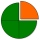Which of the following has a sum of 3/4? A. 1/2+1/4 B. 1/2+1/3 C. 1/4+1/8 D. 1/9+1/12​
• Fitness centerEvery Wednesday, Monica works out for 3/4  of an hour at the fitness center. Every Saturday, he goes to the fitness center again and exercises for 3 times as long. How much time does Wayne spend at the fitness center in all each week?
• Recipe ingredients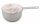Monica’s cookie recipe calls for Three-fourths of a cup of flour. Her mother’s recipe calls for Two-thirds as much as Monica’s. How many cups of flour does her mother’s recipe require?
• Antonio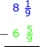Antonio is thinking of an improper fraction and a mixed fraction which have a sum of 5 5/12. The improper fraction is 8/3. What is the mixed number?
• Stones in aquariumIn an aquarium with a length of 2 m, 1.5 m wide, and 2.5 m deep, the water is up to three-quarters of the depth. Can we place 2m cubic meters of stones in the aquarium without spilling water? (0 = no, 1 = yes)
• Metal rodYou have a metal rod that’s 51/64 inches long. The rod needs to be trimmed. You cut 1/64 inches from one end and 1/32 inches from the other end. Next, you cut the rod into 6 equal pieces. What will be the final length of each piece?
• Walk for exerciseAnya, Jose, Cali, and Stephan walk for exercise. Anya's route is 2 1/4 kilometers long. Jose's route is 1 1/2 fewer km. Cali's route is 1 1/2  times as long as Jose's route, and 2 fewer km than Stephan's route. What distance (S) is Stephan's route?
• Farmer 5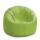Farmer Joe ordered 3 bags of soil last month. Each bag weighed 4 ⅖ kilograms. He used the first bag in a week. At the end of this month, there were 2 ¾ kilograms of soil left in the second bag and ⅞ kilograms of soil left in the third bag. How much soil wPeter bought 1/2 a pound of chocolate at rocky mountain chocolate factory. Later he went to the sweet shoppie and he bought 6/9 of a pound more chocolate. How much chocolate did he buy that day?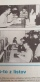There are 19 boys in section A and 23 boys in section B. If 4/7 of them are boy scout, how many boy scouts are there?A shopkeeper sells 8 1/3 kg, 10 1/4 kg and 11 1/5 kg of apples on 3 consecutive days. What is the total weight of apples sold?